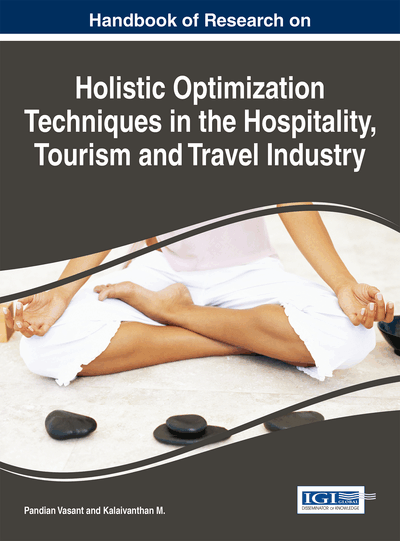# Capacity Management in Hotel Industry for Turkey

Deniz Efendioğlu (Marmara University, Turkey) and Serol Bulkan (Marmara University, Turkey)
DOI: 10.4018/978-1-5225-1054-3.ch013
Available
\$37.50
No Current Special Offers

## Abstract

The hotel industry is capital-intensive with high operating leverage in providing accommodations. Examining final costs play very important role in determining of overcapacity and undercapacity. Turkey's experiencing growth in tourism and has remarkable increases in accommodation. This situation possesses great importance for the capital groups investing in this area. How these groups and local tradesman implement a strategy on capacity management is the main question. Single period inventory model is used at the analysis phase and several interests are examined on this topic. Future Room demand is estimated by ARIMA and in estimating cost of undersupply earnings before taxes is used per room night sold. Combining the derived cost ratio with the future room demand and probability distribution estimated from the ARIMA method, the optimal hotel room capacity can be determined. The purpose of the study is to examine hotel room supply and demand for optimal capacity in Turkey.
Chapter Preview
Top

## The Single Period Inventory Model

In many situations, a decision maker encounter with the problem of determining the value of the quantity ordered of products in inventory which is shown “q”. After q has been determined, the demand is observed which is assumed by a random variable D.

## Key Terms in this Chapter

Autoregressive Moving Average (ARMA) Model: It is obtained by combining p number of autoregressive term and q number of moving average term.

Moving Average (MA) Model: It is used to describe the likelihood of a relationship between a variable and residuals obtained from the previous period.

Stationarity: ARMA (p, q) modeling process requires stability (stationary). Stationary processes do not have a certain trend and have the mean and variance that it doesn’t change with time.

Autoregressive (AR) Models: The model that the value of one period variable found depends on the value of the previous period AR(p).

ARIMA: In statistics and econometrics, and in particular in time series analysis, an autoregressive integrated moving average (ARIMA) model is a generalization of autoregressive (ARMA) model. These models are fitted to time series data either to better understand the data or to predict future points in the series (forecasting).

Autocorrelation Function (ACF) and Partial Autocorrelation Function (PACF): ACF, can be represented as the rate of autocorrelation of y t and y t-k and y t which is dependent variable. ACF (k) gives the correlation of between y t and y t-k from the raw data. Partial autocorrelation function (PACF) is actually, used to denote the simple correlation between the portions related to the delays, y t and y t-k .

Single Period Inventory Model: The single-period problem (SPP) is applied for identifying the amount of inventory to purchase given a perishable product or single opportunity. It aims to find the order quantity that maximizes expected profit by probabilistic demand distributions.

Capacity Management: Kind of a management that measuring the production capability of the business.

## Complete Chapter List

Search this Book:
Reset